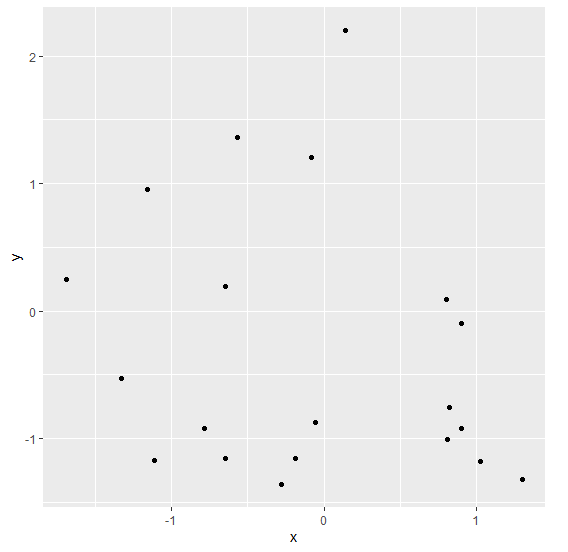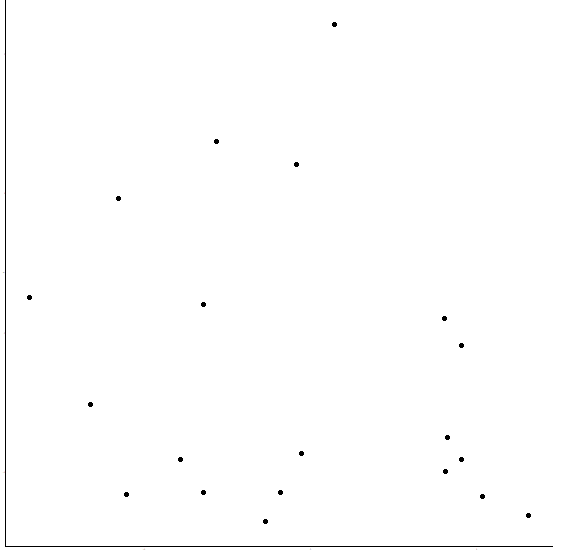# Create a ggplot2 graph without axes labels, axes titles and ticks in R.

Sometimes we want to graphs that looks like graphs on a white paper having no axes labels, axes titles, and ticks, therefore, we can use theme_classic function of ggplot2 package.

For Example, if we have a data frame called df that contains two columns say x and y then we can create the scatterplot between x and y using ggplot2 that looks like printed on white paper by using the below given command −

ggplot(df,aes(x,y))+geom_point()+theme_classic(base_size=0)

## Example

Following snippet creates a sample data frame −

x<-rnorm(20)
y<-rnorm(20)
df<-data.frame(x,y)
df


The following dataframe is created

            x           y
1 -1.32829407 -0.52533713
2  0.80283971  0.09228818
3  1.30486534 -1.32263211
4  0.82200259 -0.75838748
5 -0.78567043 -0.91903627
6 -0.64583914  0.19331685
7  0.90248805 -0.91643034
8 -0.56924149  1.36547589
9 -0.18644293 -1.15722081
10  0.90336807 -0.09976943
11  0.14253736  2.20434225
12 -1.69082324  0.24745690
13 -1.11045644 -1.17075211
14 -0.05810435 -0.87527445
15 -0.27621905 -1.36109968
16 -0.64865748 -1.15702097
17  0.81016835 -1.00659751
18 -1.15754429  0.95785839
19 -0.08498386  1.20189500
20  1.02842221 -1.18280846

To load ggplot2 package and create scatterplot between x and y on the above created data frame, add the following code to the above snippet −

x<-rnorm(20)
y<-rnorm(20)
df<-data.frame(x,y)
library(ggplot2)
ggplot(df,aes(x,y))+geom_point()

## Output

If you execute all the above given snippets as a single program, it generates the following Output −To create scatterplot between x and y just like printed on white paper on the above created data frame, add the following code to the above snippet −

x<-rnorm(20)
y<-rnorm(20)
df<-data.frame(x,y)
library(ggplot2)
ggplot(df,aes(x,y))+geom_point()+theme_classic(base_size=0)

## Output

If you execute all the above given snippets as a single program, it generates the following Output −